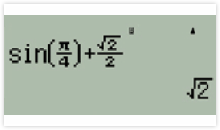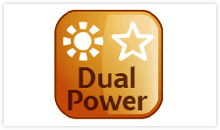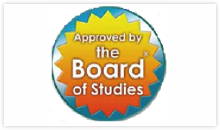FIND AUTO PARTS

 F717SGA 01With so many features and benefits the F717SGA raises the mark whether you are a teacher, student or professional. Environmentally friendly and easy-to-use, the F717SGA makes any task uncomplicated. Strong and light weight the F717SGA is made to last against the rigours of daily student life or a heavy handed workplace.
 Dual Way Textbook Display 02Featuring two types of input and display formats ('Mathematic' & 'Line') for easy scientific operations.
 Environmentally Friendly 03Manufactured using recycled Canon materials to make the top case, bottom case, battery cover and lid.
 Fast Calculation 04Advanced technologies built in to allow it to calculate complex algorithms at a fast pace, 242 functions included.
 Dual Powered - Solar 05Solar and automatic battery back-up for use anywhere, anytime.
 Easy Layout 06A familiar and easy-to-use layout used in many education facilities makes calculating quick and uncomplicated.
 Board Approved 07Fully Approved by the Board of Studies and the New Zealand Qualifications Authority.

Functions

• Internal calculation: Up to 18 digits
• Buttons/Keys: 50, including 4 way Navi key
• Scientific notation: Yes
• Engineering notation: Yes
• Memory clear: Yes &ndash; able to reset all stored memory

Environment

• Recycled Material: Yes &ndash; minimum 20% of the F717SGA is made from Canon recycled material
• Solar Powered: Yes (with auto power off after 7 minutes)

Mathematics Functions

• Addition, Subtraction, Multiplication, Division: Yes
• Square Root, Cube Root, Multiple Root: Yes
• Square, Power Square, cube: Yes
• Reciprocal: Yes
• Percentage (%): Yes
• Rounding: Yes
• Pi (Internal value = 3.14159265358979324): Yes
• Exponential e value (internal value = 2.71828182845904524): Yes
• Decimal Point selection: Yes
• Fix decimal (0-9): Yes
• Display Mode: Selectable Mathematics textbook input/output display format&nbsp;(MthIO)&nbsp;or Traditional 2-line Input/output format&nbsp;(LineIO)

Trigonometry Functions

• DRG Conversions: Yes
• Trigonometry, Inverse Trigonometry: Yes
• Hyperbolic/Inverse Hyperbolic: Yes/Yes
• Coordination Conversion (Rectangular Polar): Yes

Statistics (SD mode &amp; REG mode)

• No. of Variable:&nbsp; 2
• VAR
• Number of Samples input n: Yes
• Mean of X &amp; Y: Yes
• Standard Deviation of sample X &amp; Y: Yes
• Standard Deviation of population / parameter X &amp; Y: Yes
• MaxMin
• Median (in 1-Var Stat only): -
• Maximum Value of X &amp; Y: Yes
• Minimum Value of X &amp; Y: Yes
• SUM
• Sum of Value / Summation on X, Y: Yes
• Sum of Square of Value X2, Y2: Yes
• Sum of all XY pairs: Yes
• Sum of Cube of Value X3: Yes
• Sum of X2Y Value: Yes
• Sum of Quadrate of X-data X4: Yes
• 1-Var - Single Calculation: Yes
• 2-Var - Regression Calculation: Yes
• Linear Regression: Yes
• Quadratic Regression: Yes
• Euler Exponential Regression: Yes
• General Exponential Regression: Yes
• Logarithmic Regression: Yes
• Power Regression: Yes
• Inverse Regression: Yes
• REG
• Regression Correlation coefficient A: Yes
• Linear Regression Coefficient B: Yes
• Quadratic Coefficient C: Yes
• Estimated value of X1,X2, Y1,Y2: Yes
• Factorials (n!): Yes
• Permutations &amp; Combinations (nPr, nCr): Yes
• Random number generator (Rand): Yes
• Integer Random number generator (I~Rand): Yes

Replay

• Last Digit correction: Yes
• Last Answer recall: Yes
• Multiple replay: Yes

Other

• Hard Case: Included with convenient reference card/photo slot
• Case Colour: Available in red, blue or black
• Case Material: Upper case, Lower case, Key top &amp; Battery cover) using Anti-Bacteria Material
• Included Standard Equipment: F717SGA main unit, 1 X CR2032 backup battery, quick reference card and instruction manual
• Power Supply: Solar and battery (CR2032 replaceable)
• Low Power Indicator: Yes
 Model F717SGA Type Pocket Packing Weight 140 g Purpose Scientific Power Source Battery/Solar Digit Display 18 Digits Additional Features 242 functions 4 line dot matrix display Packing Width 200 mm Packing Depth 100 mm Packing Height 100 mm Package Type BOX

Be The First To Review This Product!

Help other Aurus Australia Pty Ltd users shop smarter by writing reviews for products you have purchased.